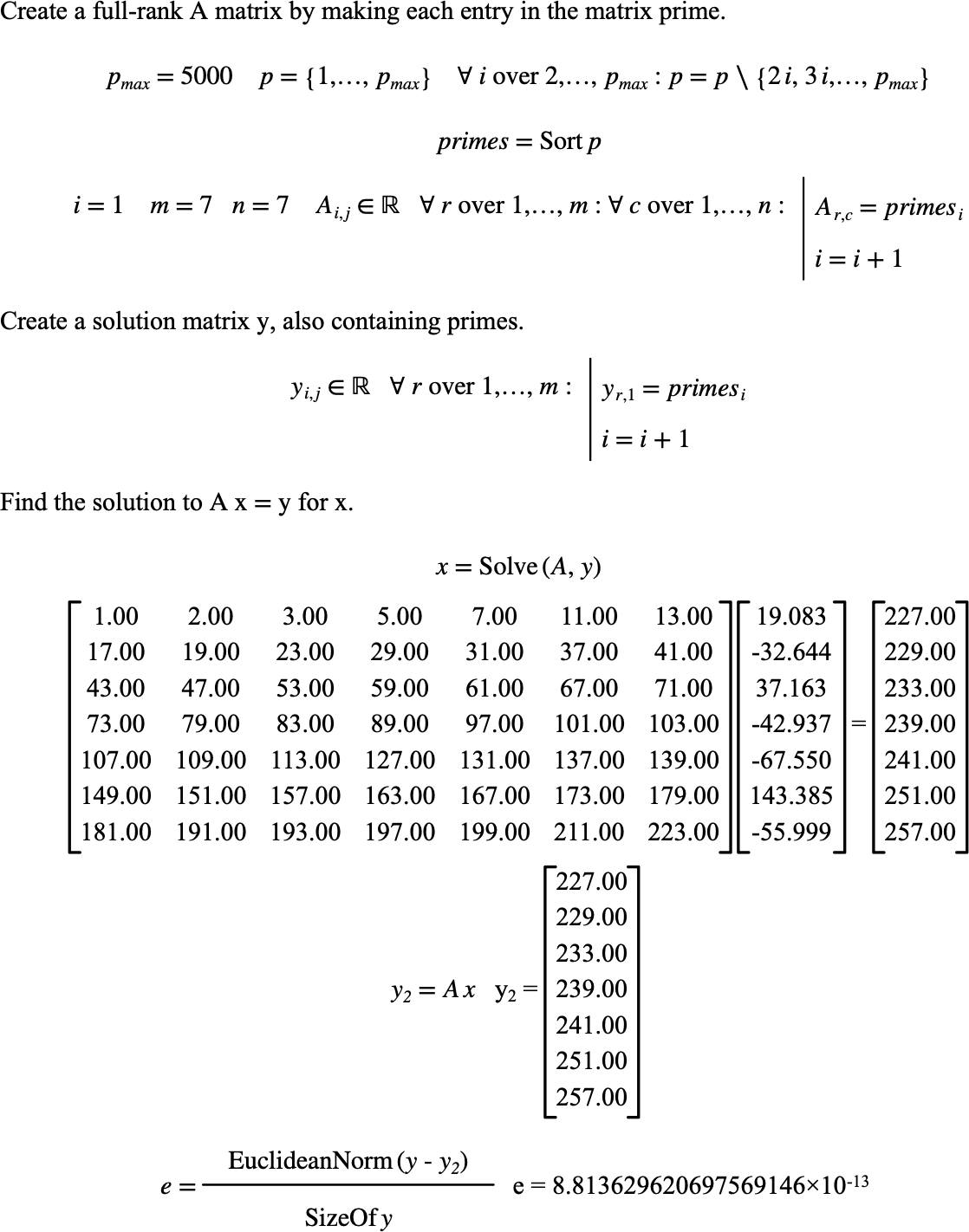# $$\text{Solve}$$¶

You can use the $$\text{Solve}$$ function to find the matrix $$x$$ given matrices $$A$$ and $$y$$ where $$A x = y$$.

You can use the \solve backslash command to insert this function.

The following variants of this function are available:

• $$\text{matrix } \text{Solve} \left ( \text{<A>}, \text{<y>} \right )$$

The $$A$$ and $$y$$ matrices represent the terms to use to solve for matrix $$x$$. Matrix $$y$$ must be a column matrix with the same number of rows in $$A$$. Matrix $$A$$ must be a square matrix.

The function will return a column matrix containing one row for each column in $$A$$.

To improve the accuracy of the solver, the $$\text{Solve}$$ function will perform an equilibration on the matrix before performing LU factorization with row pivoting to find the solution. The equilibration operation is limited to powers of 2 so as to not reduce accuracy while lowering the condition number. To improve accuracy, the $$\text{Solve}$$ will also use an iterative refinement algorithm to compute a solution.

Figure 203 shows the basic use of the $$\text{Solve}$$ function.Figure 203 Example Use Of The Solve Function